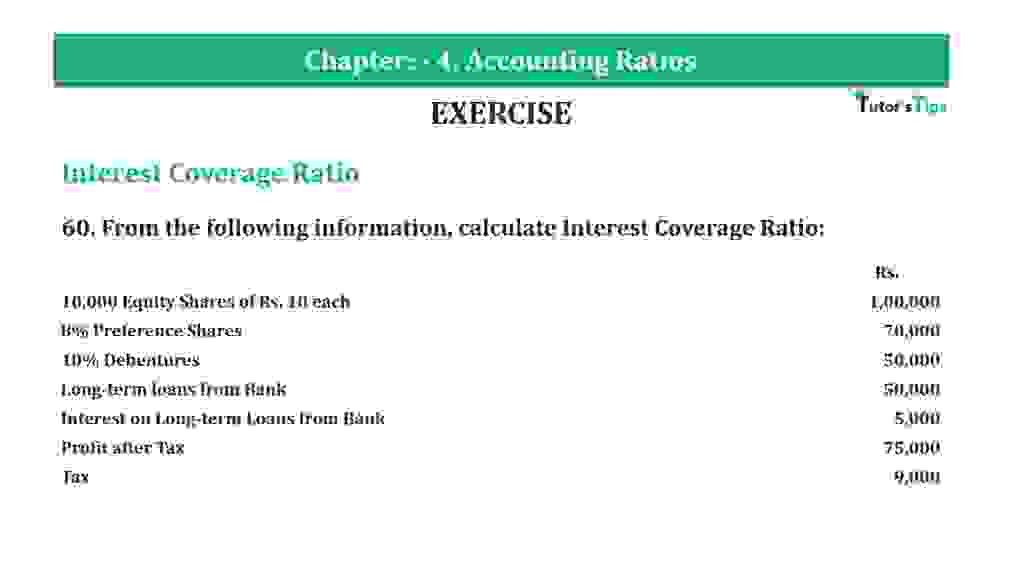# Question 60 Chapter 4 of +2-B – T.S. Grewal 12 ClassQuestion 60 Chapter 4 of +2-B

Interest Coverage Ratio

60. From the following information, calculate Interest Coverage Ratio:

 Rs. 10,000 Equity Shares of Rs. 10 each 1,00,000 8% Preference Shares 70,000 10% Debentures 50,000 Long-term loans from Bank 50,000 Interest on Long-term Loans from Bank 5,000 Profit after Tax 75,000 Tax 9,000

### The solution of Question 60 Chapter 4 of +2-B: –

 Total Interest Amount = Interest on Debentures + Interest on Long-term loans from Bank = Rs. 5,000 + Rs. 5,000 = Rs. 10,000 Profit before Interest and Tax = Profit after Tax + Tax + Interest = Rs. 75,000 + 9,000 + Rs. 5,000 +Rs. 5,000 = Rs. 94,000

 Proprietary Ratio = Net Profit before Interest and Tax = Rs.94,000 Interest Rs.10,000 = 9.4 Times

Balance Sheet: Meaning, Format & Examples

Thanks, Please Like and share with your friends

Comment if you have any question.

Also, Check out the solved question of previous Chapters: –

### T.S. Grewal’s Double Entry Book Keeping (Vol. II: Accounting for Companies)T.S. Grewal’s Analysis of Financial Statements